Ultramagic Squares of Order 7
 Samples

Special ultramagic squares
 Each of the following magic order-7 squares is ultramagic (associative and pandiagonal). Additionally it owns one or more interesting properties.

Squares with bimagic letters
 The numbers in each letter pattern (L or H) sum up to the magic constant 175. Additionally the squares of these numbers have equal sums (bimagic feature). A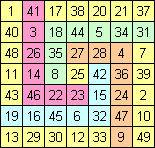B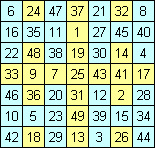C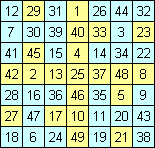Squares with miscellaneous properties
 D: The numbers in each tromino (3-cell-pattern) sum up to 3 · 25 = 75. E: The center row and the center column are 'trimagic', their numbers have equal sums, this is also true for squares of the numbers and for the third powers of the numbers. The two main diagonals are 'trimagic' too. F: The numbers in each H-pattern sum up to 175 and the bent diagonals also are magic (like Franklin's squares). For example: 9 + 26 + 40 + 25 + 3 + 44 + 28 = 175. D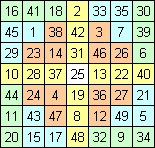E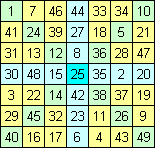F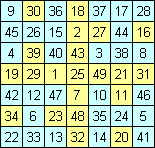Inlaid magic squares
 Within the following 6 magic squares you will find two non-normal magic 3x3-squares. Each square is shown twice with different yellow magic squares (diamonds) of order 3. G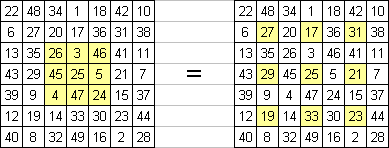J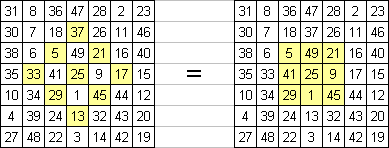H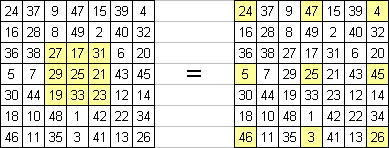K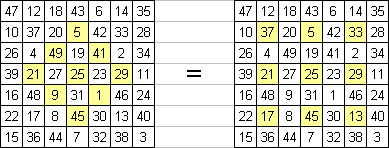I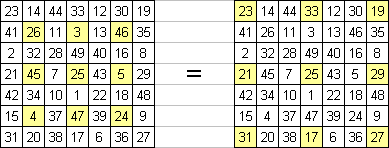L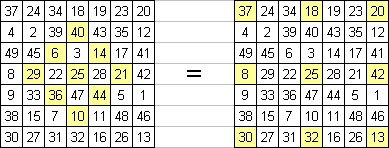Index
 summary cells equations transpositions improvements results programs files conclusions samples